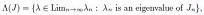##### Results on Uniqueness of Periodic Solution, Exponential Stability and Controllability Properties of Neutral Delay System

Dodi K. Igobi, Jeremiah Atsu

Journal of Advances in Mathematics and Computer Science, Page 1-20
DOI: 10.9734/JAMCS/2018/22826

The variation of constant method is employed to evaluate the periodic solution of a linear neutral system with an input function. Uniqueness of the obtained solution is established and proved by utilizing the inversion theory on a perturbed differential operator. The exponential stability of the system equation and the computation of the maximum delay bound for the system to be asymptotically stable are analyzed using the resolvent matrix of the system equation. The controllability of the system is studied by the analyses of the linear ordinary control and the free control parts of the linear neutral system for properness, non-singularity of the gramian matrix, canonical form of the controllable matrix and the non zero/ pole cancellation of the transfer function matrix. Results obtained are employed on neutral delay model of a partial element equivalent circuit (PEEC) consisting of a retarded mutual coupling between the partial inductance to confirm the suitability of the test.

##### A Heuristic Fast Gradient Descent Method for Unimodal Optimization

George Anescu

Journal of Advances in Mathematics and Computer Science, Page 1-20
DOI: 10.9734/JAMCS/2018/39798

The known gradient descent optimization methods applied to convex functions are using the gradient's magnitude in order to adaptively determine the current step size. The paper is presenting a new heuristic fast gradient descent (HFGD) approach, which uses the change in gradient's direction in order to adaptively determine the current step size. The new approach can be applied to solve classes of unimodal functions more general than the convex functions (e.g., quasi-convex functions), or as a local optimization method in multimodal optimization. Testing conducted on a testbed of 16 test functions showed an overall much better eciency and an overall better success rate of the proposed HFGD method when compared to other three known first order gradient descent methods.

##### Eigenvalues for Some Complex In nite Tridiagonal Matrices

Maria Malejki

Journal of Advances in Mathematics and Computer Science, Page 1-9
DOI: 10.9734/JAMCS/2018/39654

The discrete spectrum for an unbounded operator J dened by a special innite tridiagonal
complex matrix is approximated by the eigenvalues of its orthogonal truncations. Let σ(J) means
the spectrum of the operator J andwhere Limn→∞λn is the set of limit points of the sequence (λn); and the n x n matrix Jn is an
orthogonal truncation of J.
We consider classes of tridiagonal complex matrices for which σ(J) = Λ(J).

##### Nonlinear Sturm-Liouville Boundary Value Problems for Second Order Hamiltonian Systems with Impulsive Effects

Tingting Hu

Journal of Advances in Mathematics and Computer Science, Page 1-9
DOI: 10.9734/JAMCS/2018/39211

We investigate nontrivial solutions for nonlinear Sturm-Liouville boundary value problems of second order Hamiltonian systems with impulsive effects and we obtain some new results.

##### The First Integral Method for the Two-dimensional Incompressible Navier-Stokes Equations

Ammar Abd AL Al-Hussein

Journal of Advances in Mathematics and Computer Science, Page 1-9
DOI: 10.9734/JAMCS/2018/39070

In this paper, we deal with the first integral method to find exact solutions for The Two-Dimensional Incompressible Navier-Stokes equations. This method is an algebraic direct method used division theorem to find the first integral through polynomial and use traveling wave solution to transform the partial differential equation into the ordinary differential equation. We get different exact solutions through the use of this method and these solutions are either of the formula of exponential, hyperbolic or trigonometric functions.